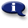# .

## Syllabus Information

Spring 2022
Dec 03,2023Use this page to maintain syllabus information, learning objectives, required materials, and technical requirements for the course.

Syllabus Information
MTH 085 - Applied Geometry for Technicians
Associated Term: Spring 2022
Learning Objectives: Upon completion of this course the student should be able to:
1. Determine angle measurements in drawings involving triangles, parallel lines, and central angles.
2. Use a protractor appropriately.
3. Name basic shapes and describe their properties.
4. Determine what folds up into a simple 3D shape.
5. Apply properties of isosceles and equilateral triangles.
6. Utilize the US Customary and metric system of units for length, area, and volume.
7. Use the US Customary and metric system of units for temperature and weight.
8. Apply unit analysis to measurements including square and cubic units.
9. Use conversion charts to convert measurements.
10. Apply densities to determine weight or volume.
11. Calculate perimeters, areas, volumes, and surface areas of geometric shapes.
12. Evaluate formulas related to geometric measure.
13. Solve formulas and simple equations for given variables.
14. Solve applied ratio and proportion problems.
15. Apply properties of similar triangles to find lengths.
16. Apply properties of right triangles and use the Pythagorean Theorem.
17. Apply trigonometric ratios to determine angles and lengths in right triangles.
18. Apply the Law of Sines and the Law of Cosines. (Optional)
19. Solve application problems involving geometry and measurement appropriate to technical fields.
20. Simplify elementary algebraic expressions.
21. Solve elementary algebraic equations and formulas.
Required Materials:
Technical Requirements: